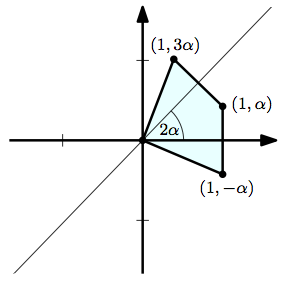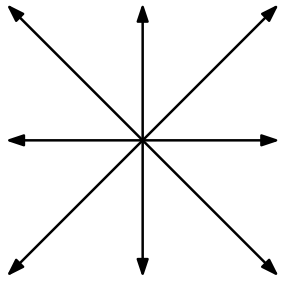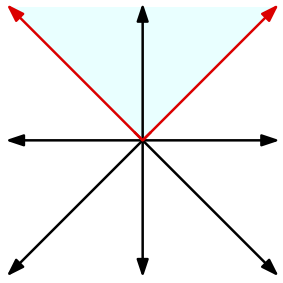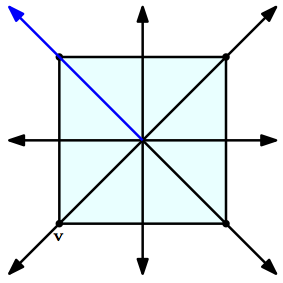# Non_matroidal matroids III

In this post I’m going to conclude my investigation of Coxeter matroids and related objects.

We can motivate the definition of Coxeter matroids by thinking about a geometrical problem in the Euclidean space $\mathbb{R}^{n}$. Recall that a (closed) half-space is a set of the form $\{\mathbf{v}\in \mathbb{R}^{n}\colon \mathbf{v}\cdot \mathbf{a}\geq b\}$, where $\mathbf{a}$ is a fixed (non-zero) vector, $b$ is a real number, and the dot denotes the standard inner product. A polyhedron is an intersection of finitely many half-spaces. A polyhedron is a polytope if it is bounded, meaning that there there must be some ball of finite radius centred on the origin that contains every point of the polytope. A vertex of a polyhedron is a point $\mathbf{p}$ in the polyhedron with the property that some affine hyperplane intersects the polyhedron exactly in $\mathbf{p}$. Similarly, an edge of a polyhedron is a line segment, $l$, such that some affine hyperplane intersects the polyhedron exactly in $l$. The terminal points of an edge are always vertices of the polytope.

Now let $P$ be a polytope. For every edge $l$ of $P$, there is an affine hyperplane that is perpendicular to $l$ and that passes through the mid-point of $l$. This affine hyperplane determines an isometry (a distance-preserving transformation) of $\mathbb{R}^{n}$: namely, reflection through the hyperplane.

What can we say about the group of isometries generated by these reflections? As the composition of any reflection with itself is the identity, this group is a Coxeter group, since the definition of such a group is that it must have a set of generators each of which has order two. When will this Coxeter group be finite? It is easy to find examples of polytopes that lead to finite Coxeter groups: just take any regular polygon. In this case the reflections generate the dihedral group. On the other hand, consider the points with polar coordinates $(0,0)$, $(1,-\alpha)$, $(1,\alpha)$, and $(1,3\alpha)$, where $\alpha$ is an irrational fraction of a full rotation. The intersection of all half-spaces that contain these four points is a polytope. The reflections corresponding to the edges of the polytope include the reflection in the horizontal axis, and also the reflection in the line with an angle of $2\alpha$ from the horizontal. Between them, these reflections generate a rotation of $4\alpha$ around the origin. The way that we chose $\alpha$ means that no finite power of this rotation will ever be equal to the identity isometry. Therefore the generated Coxeter group is infinite. The definition of Coxeter matroids is motivated by a partial solution of this problem of determining whether this generated group of isometries will be finite or infinite.We have said that any regular polygon gives rise to a finite Coxeter group of isometries.
Let us now develop another method for finding such polytopes. Let $W$ be a finite group of isometries of $\mathbb{R}^{n}$ and assume that $W$ is generated by a collection of reflections. We will show that there is a point that is fixed by every isometry in $W$. Indeed, if $\mathbf{v}\in \mathbb{R}^{n}$ is arbitrarily chosen, then the barycentre of the images of $\mathbf{v}$ is fixed by every isometry in $W$, because
$u\left(\frac{1}{|W|}\sum_{w\in W}w(\mathbf{v})\right) = \frac{1}{|W|}\sum_{w\in W}u(w(\mathbf{v})) = \frac{1}{|W|}\sum_{w\in W}w(\mathbf{v})$
for every $u\in W$. Therefore, by translating, we might as well assume that the reflections that generate $W$ all fix the origin. For each reflection $r\in W$, choose two vectors, $\mathbf{v}_{r}$ and $-\mathbf{v}_{r}$ that are orthogonal to the $r$-invariant hyperplane. Thus $r(\pm\mathbf{v}_{r})=\mp\mathbf{v}_{r}$. We shall call the collection $R=\{\pm \mathbf{v}_{r}\colon r\ \text{is a reflection in}\ W\}$ a root system of $W$. Note that the vectors in $R$ correspond in a natural way to the reflections in $W$. We have given no consideration to the length of the vectors $\mathbf{v}_{r}$, which means that we are not using the standard definition of a root system. This diagram shows a possible root system in $2$ dimensions.Now let $\mathbf{a}$ be a fixed and arbitrary vector, and let $R^{+}=\{\mathbf{u}\in R\colon \mathbf{u}\cdot\mathbf{a}>0\}$ be the set of positive roots. The positive roots generate the positive cone, consisting of all linear combinations of vectors in $R^{+}$ with all coefficients non-negative. This positive cone is a polyhedron. Those vectors in $R^{+}$ that are parallel with an edge of the positive cone form a simple system of roots. In the following diagram, we have chosen $\mathbf{a}$ to be the vector $(1,0)$. There are three positive roots, and they generate the shaded positive cone. The two vectors coloured red form the corresponding simple system.Let $I$ be the simple system of roots, and let $J$ be a non-empty subset of $I$. The roots in $J$ correspond to a collection of reflections in $W$. Let $P$ be the subgroup of $W$ generated by these reflections. This subgroup is called a standard parabolic subgroup of $W$. In our example, let $P$ be the subgroup generated by the reflection along the line parallel to $(1,1)$, that is, the reflection corresponding to the blue vector in the diagram below. Let $\mathcal{M}$ be a collection of cosets of $P$. In our example, we will keep things simple, and just let $\mathcal{M}$ be the collection of all cosets. We choose a point $\mathbf{v}$ that is fixed by $P$. For every coset $wP$ in $\mathcal{M}$, we find the image of $\mathbf{v}$ under $w$. Note that this image is well-defined, in the sense that it does not depend on the representative $w$, since $\mathbf{v}$ will be fixed by every isometry in $P$. Now we have generated a collection of images of $\mathbf{v}$. We construct the convex hull of these images: that is, we take the polytope consisting of the intersection of all half-spaces that contain the images of $\mathbf{v}$. It turns out that the Coxeter group generated by the edges of this polytope is finite (a subgroup of $W$ in fact). In the diagram below, the vector $\mathbf{v}$ is marked. There are four images of $\mathbf{v}$ under cosets of $P$, and the convex hull of those images is the shaded square.What is surprising about this construction, is that every polytope generating a finite Coxeter group can essentially be constructed in this way. This is a consequence of a theorem due to Gelfand and Serganova (details are in the book Coxeter matroids).
So far, the link to matroids is not at all obvious, so I will conclude by drawing a connection back to my first post. Let $w_{1},\ldots, w_{t}$ be the reflections in $W$ that correspond to the vectors in a simple root system. Then $W$ is generated by $w_{1},\ldots, w_{t}$, so every $u\in W$ can be expressed as a word in these reflections. Such a word is said to be reduced if it is as short as possible. Now for $u,v\in W$ we say that $v\leq u$ in the Bruhat ordering if there is a reduced word that represents $u$, with the property that I can obtain a word representing $v$ by deleting some characters. If $w\in W$, then we write $v\leq^{w} u$ to mean $w^{-1}v\leq w^{-1}u$. If $A$ and $B$ are cosets, then $A\leq^{w} B$ means that $v\leq^{w} u$ for some representatives $v\in A$ and $u\in B$. The connection to matroids comes from the collection $\mathcal{M}$ of cosets. In our construction, $\mathcal{M}$ has the following property: if $w$ is any reflection in $W$, then there is a coset $B\in \mathcal{M}$ with the property that $A\leq^{w} B$ for all $A\in \mathcal{M}$. Now the resemblance to matroids, as axiomatised via Gale’s Theorem, is quite striking. The cosets in $\mathcal{M}$ play the role of bases in Gale’s Theorem. Now we can finally give the definition of a Coxeter matroid, which is justified by this resemblance to Gale’s axiomatization. If $W$ is a Coxeter group with $P$ as a parabolic subgroup, and $\mathcal{M}$ is a collection of cosets, then $\mathcal{M}$ is a Coxeter matroid if, for every $w\in W$, there is a coset $B\in \mathcal{M}$ such that $A\leq^{w} B$ for all $A\in \mathcal{M}$.

This site uses Akismet to reduce spam. Learn how your comment data is processed.